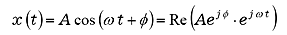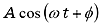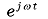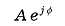FFT analyzer FAQWhat is a Fourier spectrum?A Fourier spectrum refers to the result that is obtained by processing a time signal with Fourier conversion and is expressed in the frequency function format. However, under the FFT analyzer, the Fourier series that is obtained by expanding a complex Fourier series assuming the finite time window as the basic cycle is referred to as a Fourier spectrum. Therefore, if the physical unit of the time signal is EU(V), the unit of the absolute value of the Fourier spectrum is also expressed as EU(V).This concept is expressed in a complex number by formula (1), assuming the input signal asRe indicates the real part of the complex number. Sincerefers to the vector (ω =2πf, component of frequency f) that rotates on the unit circle anticlockwise at the angle speed (angle frequency), the Fourier spectrum under frequency f becomes complex amplitude () where t = 0 in expression (1). A and φ refer to the size (mag) and the phase of the Fourier spectrum respectively.

Revised:2009/11/16